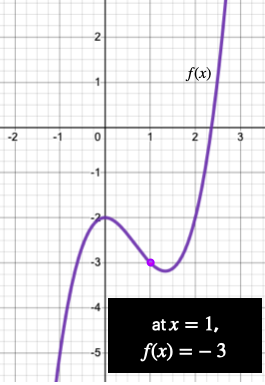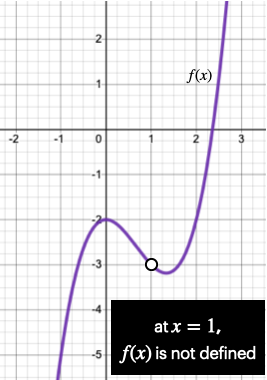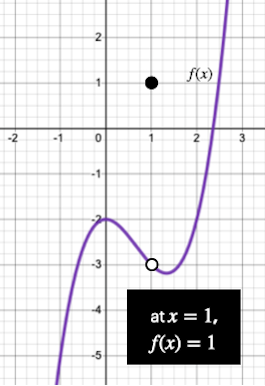407 493 6601

# Precalculus - Introductions to Limits

## Introduction

• The limit of a function describes the function's behavior close to a point.
• In mathematical terms, a limit is expressed as $\underset{x\to a}{\mathrm{lim}}f\left(x\right)=L$, which means that as $x$ "approaches" a real value $a$, the function $f\left(x\right)$ "approaches" a real value $L$.
• This does not necessarily mean that the value of the function $f\left(x\right)$ at $x=a$ is $L$.
• In fact, there can be three possibilities, as shown in the figures below.
• Value of $f\left(x\right)$ at $x=a$ is $L$• Value of $f\left(x\right)$ at $x=a$ does not exist (not defined)• Value of $f\left(x\right)$ at $x=a$ is some value other than $L$## Properties of Limits

• Sum Rule: The limit of the sum of two functions is the sum of their individual limits.

$\underset{x\to a}{\mathrm{lim}}\left[f\left(x\right)+g\left(x\right)\right]=\underset{x\to a}{\mathrm{lim}}f\left(x\right)+\underset{x\to a}{\mathrm{lim}}g\left(x\right)$

• Difference Rule: The limit of the difference between two functions is the difference between their individual limits.

$\underset{x\to a}{\mathrm{lim}}\left[f\left(x\right)-g\left(x\right)\right]=\underset{x\to a}{\mathrm{lim}}f\left(x\right)-\underset{x\to a}{\mathrm{lim}}g\left(x\right)$

• Product of two functions Rule: The limit of a product of two functions is the product of their individual limits.

$\underset{x\to a}{\mathrm{lim}}\left[f\left(x\right)·g\left(x\right)\right]=\underset{x\to a}{\mathrm{lim}}f\left(x\right)·\underset{x\to a}{\mathrm{lim}}g\left(x\right)$

• Product of a function with a constant Rule: The limit of constant times a function is the constant times the limit of the function.

$\underset{x\to a}{\mathrm{lim}}\left[k·f\left(x\right)\right]=k·\underset{x\to a}{\mathrm{lim}}f\left(x\right)$

• Division Rule: The limit of a quotient of two functions is the quotient of their limits, provided the limit of the denominator is not zero.

$\underset{x\to a}{\mathrm{lim}}\left[\frac{f\left(x\right)}{g\left(x\right)}\right]=\frac{\underset{x\to a}{\mathrm{lim}}f\left(x\right)}{\underset{x\to a}{\mathrm{lim}}g\left(x\right)}$

## Evaluating Limits

There are various techniques for evaluating the limits of functions. Some of the most commonly used methods are described below.

• Direct Substitution Method: In this method, we simply put the value of the limit in the given function.

For example, $\underset{x\to 2}{\mathrm{lim}}\frac{x-3}{2x}$$=\frac{\left(2\right)-3}{2\left(2\right)}$$=\frac{-1}{4}$

Sometimes, the direct substitution method results in an indeterminate form. Such limits are evaluated using the techniques mentioned below.

• Factorization Method: This method is generally used to find the limits of rational functions. In factorization, we factor both the numerator and denominator of the function and cancel the common factors that make the denominator equal to zero.

For example, $\underset{x\to 1}{\mathrm{lim}}\frac{{x}^{2}+x-2}{x-1}$

$=\underset{x\to 1}{\mathrm{lim}}\frac{\left(x-1\right)\left(x+2\right)}{\left(x-1\right)}$

$=\underset{x\to 1}{\mathrm{lim}}\left(x+2\right)$$=1+2$$=3$

• Rationalization Method: This method is used to find the limits of functions with fractional powers (with functions that contain radical expressions).

For example, $\underset{x\to 2}{\mathrm{lim}}\frac{3-\sqrt{x+7}}{x-2}$

$=\underset{x\to 2}{\mathrm{lim}}\frac{3-\sqrt{x+7}}{x-2}×\frac{3+\sqrt{x+7}}{3+\sqrt{x+7}}$

$=\underset{x\to 2}{\mathrm{lim}}\frac{9-\left(x+7\right)}{\left(x-2\right)\left(3+\sqrt{x+7}\right)}$

$=\underset{x\to 2}{\mathrm{lim}}\frac{9-x-7}{\left(x-2\right)\left(3+\sqrt{x+7}\right)}$

$=\underset{x\to 2}{\mathrm{lim}}\frac{\left(2-x\right)}{\left(x-2\right)\left(3+\sqrt{x+7}\right)}$

$=\underset{x\to 2}{\mathrm{lim}}\frac{-\left(x-2\right)}{\left(x-2\right)\left(3+\sqrt{x+7}\right)}$

$=\underset{x\to 2}{\mathrm{lim}}\frac{-1}{\left(3+\sqrt{x+7}\right)}$

$=\frac{-1}{\left(3+\sqrt{9}\right)}$

$=\frac{-1}{\left(3+3\right)}$

$=-\frac{1}{6}$

• Reducing to standard forms: In this method, we reduce the given limit to one of the standard forms whose limits are known to us, as mentioned below.
• $\underset{x\to a}{\mathrm{lim}}\frac{{x}^{n}-{a}^{n}}{x-a}=n{a}^{n-1}$
• $\underset{x\to \infty }{\mathrm{lim}}{\left(1+\frac{a}{x}\right)}^{x}={e}^{a}$
• $\underset{x\to 0}{\mathrm{lim}}{\left(1+x\right)}^{1}{x}}=e$
• $\underset{x\to 0}{\mathrm{lim}}\frac{{e}^{x}-1}{x}=1$
• L'Hospital's Rule: This rule is used to evaluate limits of indeterminate form such as $\frac{0}{0}$$\frac{\infty }{\infty }$$\infty -\infty$${0}^{0}$${1}^{\infty }$, and ${\infty }^{0}$.

This rule states that if $\underset{x\to a}{\mathrm{lim}}\frac{f\left(x\right)}{g\left(x\right)}$ results in one of the indeterminate forms mentioned above, then $\underset{x\to a}{\mathrm{lim}}\frac{f\left(x\right)}{g\left(x\right)}=\underset{x\to a}{\mathrm{lim}}\frac{{f}^{\text{'}}\left(x\right)}{{g}^{\text{'}}\left(x\right)}$, provided $\underset{x\to a}{\mathrm{lim}}\frac{{f}^{\text{'}}\left(x\right)}{{g}^{\text{'}}\left(x\right)}$ exists and ${g}^{\text{'}}\left(x\right)\ne 0$.

## Solved Examples

Example 1: Evaluate $\underset{x\to -1}{\mathrm{lim}}\left(5-3x\right)$.

Solution: $\underset{x\to -1}{\mathrm{lim}}\left(5-3x\right)$

$=5-3\left(-1\right)$ (Direct Substitution Method)

$=5+3$

$=8$

Example 2: Evaluate $\underset{x\to 1}{\mathrm{lim}}\frac{2{x}^{2}-5x+3}{{x}^{2}+x-2}$.

Solution: $\underset{x\to 1}{\mathrm{lim}}\frac{2{x}^{2}-5x+3}{{x}^{2}+x-2}$

$=\frac{2{\left(1\right)}^{2}-5\left(1\right)+3}{{\left(1\right)}^{2}+\left(1\right)-2}$$=\frac{2-5+3}{1+1-2}$$=\frac{0}{0}$

$=\underset{x\to 1}{\mathrm{lim}}\frac{\left(2x-3\right)\left(x-1\right)}{\left(x-1\right)\left(x+2\right)}$ (Factorization Method)

$=\underset{x\to 1}{\mathrm{lim}}\frac{\left(2x-3\right)}{\left(x+2\right)}$

$=\frac{2\left(1\right)-3}{1+2}$

$=\frac{-1}{3}$

## Cheat Sheet

• The limit of a function $f\left(x\right)$ describes the function's behavior close to a point $x=a$ and is mathematically represented as $\underset{x\to a}{\mathrm{lim}}f\left(x\right)=L$.
• Simple limits (determinate form) of functions are evaluated by the direct substitution method.
• Limits involving indeterminate forms are evaluated using techniques such as factorization, rationalization, and L'Hospital's rule.

## Blunder Areas

• For a function $f\left(x\right)$ and a real number $a$$\underset{x\to a}{\mathrm{lim}}f\left(x\right)$ and $f\left(a\right)$ may not be the same.